# Speaker Verification Using Gaussian Mixture Model

Speaker verification, or authentication, is the task of verifying that a given speech segment belongs to a given speaker. In speaker verification systems, there is an unknown set of all other speakers, so the likelihood that an utterance belongs to the verification target is compared to the likelihood that it does not. This contrasts with speaker identification tasks, where the likelihood of each speaker is calculated, and those likelihoods are compared. Both speaker verification and speaker identification can be text dependent or text independent. In this example, you create a text-dependent speaker verification system using a Gaussian mixture model/universal background model (GMM-UBM).

A sketch of the GMM-UBM system is shown: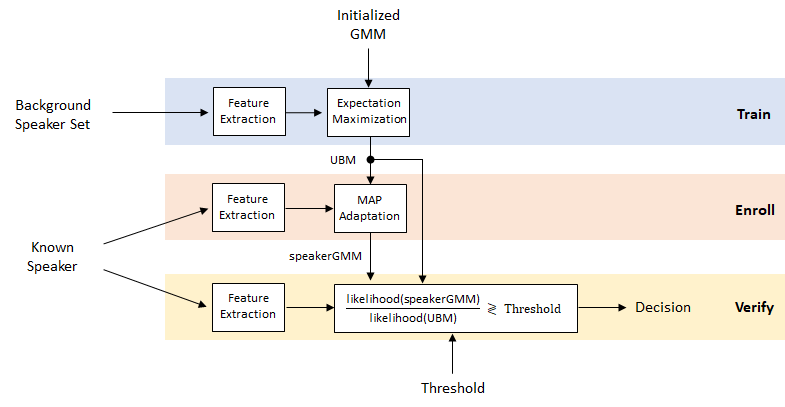### Perform Speaker Verification

To motivate this example, you will first perform speaker verification using a pre-trained universal background model (UBM). The model was trained using the word "stop" from the Google Speech Commands data set .

The MAT file, `speakerVerficationExampleData.mat`, includes the UBM, a configured `audioFeatureExtractor` object, and normalization factors used to normalize the features.

`load speakerVerificationExampleData.mat ubm afe normFactors`

### Enroll

If you would like to test enrolling yourself, set `enrollYourself` to `true`. You will be prompted to record yourself saying "stop" several times. Say "stop" only once per prompt. Increasing the number of recordings should increase the verification accuracy.

```enrollYourself =false; if enrollYourself numToRecord =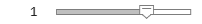5; ID =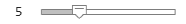'self'; helperAddUser(afe.SampleRate,numToRecord,ID); end```

Create an `audioDatastore` object to point to the five audio files included with this example, and, if you enrolled yourself, the audio files you just recorded. The audio files included with this example are part of an internally created data set and were not used to train the UBM.

`ads = audioDatastore(pwd);`

The files included with this example consist of the word "stop" spoken five times by three different speakers: `BFn` (1), `BHm` (3), and `RPalanim` (1). The file names are in the format SpeakerID_RecordingNumber. Set the datastore labels to the corresponding speaker ID.

```[~,fileName] = cellfun(@(x)fileparts(x),ads.Files,'UniformOutput',false); fileName = split(fileName,'_'); speaker = strcat(fileName(:,1)); ads.Labels = categorical(speaker);```

Use all but one file from the speaker you are enrolling for the enrollment process. The remaining files are used to test the system.

```if enrollYourself enrollLabel = ID; else enrollLabel = 'BHm'; end forEnrollment = ads.Labels==enrollLabel; forEnrollment(find(forEnrollment==1,1)) = false; adsEnroll = subset(ads,forEnrollment); adsTest = subset(ads,~forEnrollment);```

Enroll the chosen speaker using maximum a posteriori (MAP) adaptation. You can find details of the enrollment algorithm later in the example.

`speakerGMM = helperEnroll(ubm,afe,normFactors,adsEnroll);`

### Verification

For each of the files in the test set, use the likelihood ratio test and a threshold to determine whether the speaker is the enrolled speaker or an imposter.

```threshold =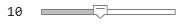0.1; reset(adsTest) while hasdata(adsTest) fprintf('Identity to confirm: %s\n',enrollLabel) [audioData,adsInfo] = read(adsTest); fprintf(' | Speaker identity: %s\n',string(adsInfo.Label)) verificationStatus = helperVerify(audioData,afe,normFactors,speakerGMM,ubm,threshold); if verificationStatus fprintf(' | Confirmed.\n'); else fprintf(' | Imposter!\n'); end end```
```Identity to confirm: BHm | Speaker identity: BFn | Imposter! Identity to confirm: BHm | Speaker identity: BHm | Confirmed. Identity to confirm: BHm | Speaker identity: RPalanim | Imposter! ```

The remainder of the example details the creation of the UBM and the enrollment algorithm, and then evaluates the system using commonly reported metrics.

### Create Universal Background Model

The UBM used in this example is trained using . Download and extract the data set.

```url = 'https://storage.googleapis.com/download.tensorflow.org/data/speech_commands_v0.01.tar.gz'; downloadFolder = tempdir; datasetFolder = fullfile(downloadFolder,'google_speech'); if ~exist(datasetFolder,'dir') disp('Downloading Google speech commands data set (1.9 GB)...') untar(url,datasetFolder) end```

Create an `audioDatastore` that points to the dataset. Use the folder names as the labels. The folder names indicate the words spoken in the dataset.

`ads = audioDatastore(datasetFolder,"Includesubfolders",true,'LabelSource','folderNames');`

`subset` the dataset to only include the word "stop".

`ads = subset(ads,ads.Labels==categorical("stop"));`

Set the labels to the unique speaker IDs encoded in the file names. The speaker IDs sometimes start with a number: add an `'a'` to all the IDs to make the names more variable friendly.

```[~,fileName] = cellfun(@(x)fileparts(x),ads.Files,'UniformOutput',false); fileName = split(fileName,'_'); speaker = strcat('a',fileName(:,1)); ads.Labels = categorical(speaker);```

Create three datastores: one for enrollment, one for evaluating the verification system, and one for training the UBM. Enroll speakers who have at least three utterances. For each of the speakers, place two of the utterances in the enrollment set. The others will go in the test set. The test set consists of utterances from all speakers who have three or more utterances in the dataset. The UBM training set consists of the remaining utterances.

```numSpeakersToEnroll =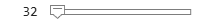10; labelCount = countEachLabel(ads); forEnrollAndTestSet = labelCount{:,1}(labelCount{:,2}>=3); forEnroll = forEnrollAndTestSet(randi([1,numel(forEnrollAndTestSet)],numSpeakersToEnroll,1)); tf = ismember(ads.Labels,forEnroll); adsEnrollAndValidate = subset(ads,tf); adsEnroll = splitEachLabel(adsEnrollAndValidate,2); adsTest = subset(ads,ismember(ads.Labels,forEnrollAndTestSet)); adsTest = subset(adsTest,~ismember(adsTest.Files,adsEnroll.Files)); forUBMTraining = ~(ismember(ads.Files,adsTest.Files) | ismember(ads.Files,adsEnroll.Files)); adsTrainUBM = subset(ads,forUBMTraining);```

Read from the training datastore and listen to a file. Reset the datastore.

```[audioData,audioInfo] = read(adsTrainUBM); fs = audioInfo.SampleRate; sound(audioData,fs) reset(adsTrainUBM)```

#### Feature Extraction

In the feature extraction pipeline for this example, you:

1. Normalize the audio

2. Use `detectSpeech` to remove nonspeech regions from the audio

3. Extract features from the audio

4. Normalize the features

5. Apply cepstral mean normalization

First, create an `audioFeatureExtractor` object to extract the MFCC. Specify a 40 ms duration and 10 ms hop for the frames.

```windowDuration = 0.04; hopDuration = 0.01; windowSamples = round(windowDuration*fs); hopSamples = round(hopDuration*fs); overlapSamples = windowSamples - hopSamples; afe = audioFeatureExtractor( ... 'SampleRate',fs, ... 'Window',hann(windowSamples,'periodic'), ... 'OverlapLength',overlapSamples, ... ... 'mfcc',true);```

Normalize the audio.

`audioData = audioData./max(abs(audioData));`

Use the `detectSpeech` function to locate the region of speech in the audio clip. Call `detectSpeech` without any output arguments to visualize the detected region of speech.

`detectSpeech(audioData,fs);`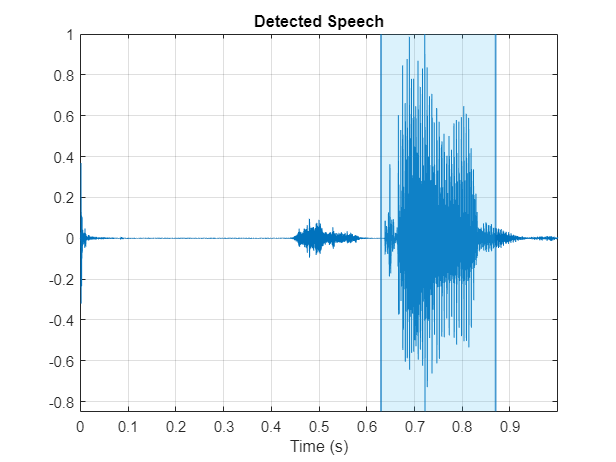Call `detectSpeech` again. This time, return the indices of the speech region and use them to remove nonspeech regions from the audio clip.

```idx = detectSpeech(audioData,fs); audioData = audioData(idx(1,1):idx(1,2));```

Call `extract` on the `audioFeatureExtractor` object to extract features from audio data. The size output from `extract` is `numHops`-by-`numFeatures`.

```features = extract(afe,audioData); [numHops,numFeatures] = size(features)```
```numHops = 66 ```
```numFeatures = 13 ```

Normalize the features by their global mean and variance. The next section of the example walks through calculating the global mean and variance. For now, just use the precalculated mean and variance already loaded.

`features = (features' - normFactors.Mean) ./ normFactors.Variance;`

Apply a local cepstral mean normalization.

`features = features - mean(features,'all');`

The feature extraction pipeline is encapsulated in the helper function, helperFeatureExtraction.

#### Calculate Global Feature Normalization Factors

Extract all features from the data set. If you have the Parallel Computing Toolbox™, determine the optimal number of partitions for the dataset and spread the computation across available workers. If you do not have Parallel Computing Toolbox™, use a single partition.

```featuresAll = {}; if ~isempty(ver('parallel')) pool = gcp; numPar = numpartitions(ads,pool); else numPar = 1; end```

Use the helper function, `helperFeatureExtraction`, to extract all features from the dataset. Calling `helperFeatureExtraction` with an empty third argument performs the feature extraction steps described in Feature Extraction except for the normalization by global mean and variance.

```parfor ii = 1:numPar adsPart = partition(ads,numPar,ii); featuresPart = cell(0,numel(adsPart.Files)); for iii = 1:numel(adsPart.Files) audioData = read(adsPart); featuresPart{iii} = helperFeatureExtraction(audioData,afe,[]); end featuresAll = [featuresAll,featuresPart]; end allFeatures = cat(2,featuresAll{:});```

Calculate the mean and variance of each feature.

```normFactors.Mean = mean(allFeatures,2,'omitnan'); normFactors.STD = std(allFeatures,[],2,'omitnan');```

#### Initialize GMM

The universal background model is a Gaussian mixture model. Define the number of components in the mixture.  suggests more than 512 for text-independent systems. The component weights begin evenly distributed.

```numComponents =32; alpha = ones(1,numComponents)/numComponents;```

Use random initialization for the `mu` and `sigma` of each GMM component. Create a structure to hold the necessary UBM information.

```mu = randn(numFeatures,numComponents); sigma = rand(numFeatures,numComponents); ubm = struct('ComponentProportion',alpha,'mu',mu,'sigma',sigma);```

#### Train UBM Using Expectation-Maximization

Fit the GMM to the training set to create the UBM. Use the expectation-maximization algorithm.

The expectation-maximization algorithm is recursive. First, define the stopping criteria.

```maxIter = 20; targetLogLikelihood = 0; tol = 0.005; pastL = -inf; % initialization of previous log-likelihood```

In a loop, train the UBM using the expectation-maximization algorithm.

```tic for iter = 1:maxIter % EXPECTATION N = zeros(1,numComponents); F = zeros(numFeatures,numComponents); S = zeros(numFeatures,numComponents); L = 0; for ii = 1:numPar adsPart = partition(adsTrainUBM,numPar,ii); while hasdata(adsPart) audioData = read(adsPart); % Extract features features = helperFeatureExtraction(audioData,afe,normFactors); % Compute a posteriori log-likelihood logLikelihood = helperGMMLogLikelihood(features,ubm); % Compute a posteriori normalized probability logLikelihoodSum = helperLogSumExp(logLikelihood); gamma = exp(logLikelihood - logLikelihoodSum)'; % Compute Baum-Welch statistics n = sum(gamma,1); f = features * gamma; s = (features.*features) * gamma; % Update the sufficient statistics over utterances N = N + n; F = F + f; S = S + s; % Update the log-likelihood L = L + sum(logLikelihoodSum); end end % Print current log-likelihood and stop if it meets criteria. L = L/numel(adsTrainUBM.Files); fprintf('\tIteration %d, Log-likelihood = %0.3f\n',iter,L) if L > targetLogLikelihood || abs(pastL - L) < tol break else pastL = L; end % MAXIMIZATION N = max(N,eps); ubm.ComponentProportion = max(N/sum(N),eps); ubm.ComponentProportion = ubm.ComponentProportion/sum(ubm.ComponentProportion); ubm.mu = bsxfun(@rdivide,F,N); ubm.sigma = max(bsxfun(@rdivide,S,N) - ubm.mu.^2,eps); end```
``` Iteration 1, Log-likelihood = -831.395 Iteration 2, Log-likelihood = -526.217 Iteration 3, Log-likelihood = -489.717 Iteration 4, Log-likelihood = -478.680 Iteration 5, Log-likelihood = -472.411 Iteration 6, Log-likelihood = -468.099 Iteration 7, Log-likelihood = -465.051 Iteration 8, Log-likelihood = -462.790 Iteration 9, Log-likelihood = -460.845 Iteration 10, Log-likelihood = -459.270 Iteration 11, Log-likelihood = -458.023 Iteration 12, Log-likelihood = -457.047 Iteration 13, Log-likelihood = -456.238 Iteration 14, Log-likelihood = -455.571 Iteration 15, Log-likelihood = -455.037 Iteration 16, Log-likelihood = -454.579 Iteration 17, Log-likelihood = -454.206 Iteration 18, Log-likelihood = -453.876 Iteration 19, Log-likelihood = -453.562 Iteration 20, Log-likelihood = -453.229 ```
`fprintf('UBM training completed in %0.2f seconds.\n',toc)`
```UBM training completed in 187.69 seconds. ```

### Enrollment: Maximum a Posteriori (MAP) Estimation

Once you have a universal background model, you can enroll speakers and adapt the UBM to the speakers.  suggests an adaptation relevance factor of 16. The relevance factor controls how much to move each component of the UBM to the speaker GMM.

```relevanceFactor = 16; speakers = unique(adsEnroll.Labels); numSpeakers = numel(speakers); gmmCellArray = cell(numSpeakers,1); tic parfor ii = 1:numSpeakers % Subset the datastore to the speaker you are adapting. adsTrainSubset = subset(adsEnroll,adsEnroll.Labels==speakers(ii)); N = zeros(1,numComponents); F = zeros(numFeatures,numComponents); S = zeros(numFeatures,numComponents); while hasdata(adsTrainSubset) audioData = read(adsTrainSubset); features = helperFeatureExtraction(audioData,afe,normFactors); [n,f,s,l] = helperExpectation(features,ubm); N = N + n; F = F + f; S = S + s; end % Determine the maximum likelihood gmm = helperMaximization(N,F,S); % Determine adaption coefficient alpha = N ./ (N + relevanceFactor); % Adapt the means gmm.mu = alpha.*gmm.mu + (1-alpha).*ubm.mu; % Adapt the variances gmm.sigma = alpha.*(S./N) + (1-alpha).*(ubm.sigma + ubm.mu.^2) - gmm.mu.^2; gmm.sigma = max(gmm.sigma,eps); % Adapt the weights gmm.ComponentProportion = alpha.*(N/sum(N)) + (1-alpha).*ubm.ComponentProportion; gmm.ComponentProportion = gmm.ComponentProportion./sum(gmm.ComponentProportion); gmmCellArray{ii} = gmm; end fprintf('Enrollment completed in %0.2f seconds.\n',toc)```
```Enrollment completed in 0.18 seconds. ```

For bookkeeping purposes, convert the cell array of GMMs to a struct, with the fields being the speaker IDs and the values being the GMM structs.

```for i = 1:numel(gmmCellArray) enrolledGMMs.(string(speakers(i))) = gmmCellArray{i}; end```

### Evaluation

#### Speaker False Rejection Rate

The speaker false rejection rate (FRR) is the rate that a given speaker is incorrectly rejected. Use the known speaker set to determine the speaker false rejection rate for a set of thresholds.

```speakers = unique(adsEnroll.Labels); numSpeakers = numel(speakers); llr = cell(numSpeakers,1); tic parfor speakerIdx = 1:numSpeakers localGMM = enrolledGMMs.(string(speakers(speakerIdx))); adsTestSubset = subset(adsTest,adsTest.Labels==speakers(speakerIdx)); llrPerSpeaker = zeros(numel(adsTestSubset.Files),1); for fileIdx = 1:numel(adsTestSubset.Files) audioData = read(adsTestSubset); [x,numFrames] = helperFeatureExtraction(audioData,afe,normFactors); logLikelihood = helperGMMLogLikelihood(x,localGMM); Lspeaker = helperLogSumExp(logLikelihood); logLikelihood = helperGMMLogLikelihood(x,ubm); Lubm = helperLogSumExp(logLikelihood); llrPerSpeaker(fileIdx) = mean(movmedian(Lspeaker - Lubm,3)); end llr{speakerIdx} = llrPerSpeaker; end fprintf('False rejection rate computed in %0.2f seconds.\n',toc)```
```False rejection rate computed in 0.17 seconds. ```

Plot the false rejection rate as a function of the threshold.

```llr = cat(1,llr{:}); thresholds = -0.5:0.01:2.5; FRR = mean(llr<thresholds); plot(thresholds,FRR*100) title('False Rejection Rate (FRR)') xlabel('Threshold') ylabel('Incorrectly Rejected (%)') grid on```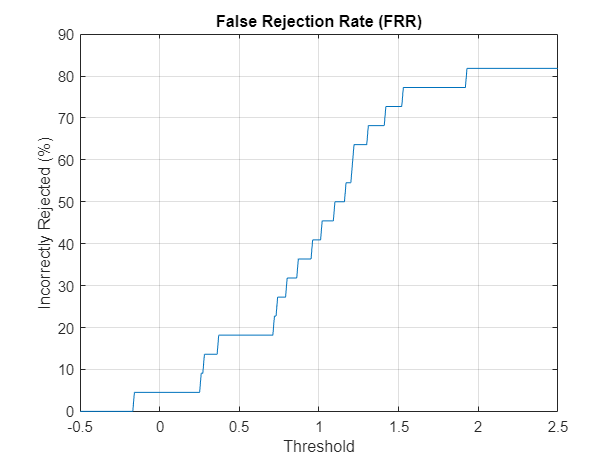#### Speaker False Acceptance

The speaker false acceptance rate (FAR) is the rate that utterances not belonging to an enrolled speaker are incorrectly accepted as belonging to the enrolled speaker. Use the known speaker set to determine the speaker FAR for a set of thresholds. Use the same set of thresholds used to determine FRR.

```speakersTest = unique(adsTest.Labels); llr = cell(numSpeakers,1); tic parfor speakerIdx = 1:numel(speakers) localGMM = enrolledGMMs.(string(speakers(speakerIdx))); adsTestSubset = subset(adsTest,adsTest.Labels~=speakers(speakerIdx)); llrPerSpeaker = zeros(numel(adsTestSubset.Files),1); for fileIdx = 1:numel(adsTestSubset.Files) audioData = read(adsTestSubset); [x,numFrames] = helperFeatureExtraction(audioData,afe,normFactors); logLikelihood = helperGMMLogLikelihood(x,localGMM); Lspeaker = helperLogSumExp(logLikelihood); logLikelihood = helperGMMLogLikelihood(x,ubm); Lubm = helperLogSumExp(logLikelihood); llrPerSpeaker(fileIdx) = mean(movmedian(Lspeaker - Lubm,3)); end llr{speakerIdx} = llrPerSpeaker; end fprintf('FAR computed in %0.2f seconds.\n',toc)```
```FAR computed in 19.66 seconds. ```

Plot the FAR as a function of the threshold.

```llr = cat(1,llr{:}); FAR = mean(llr>thresholds); plot(thresholds,FAR*100) title('False Acceptance Rate (FAR)') xlabel('Threshold') ylabel('Incorrectly Rejected (%)') grid on```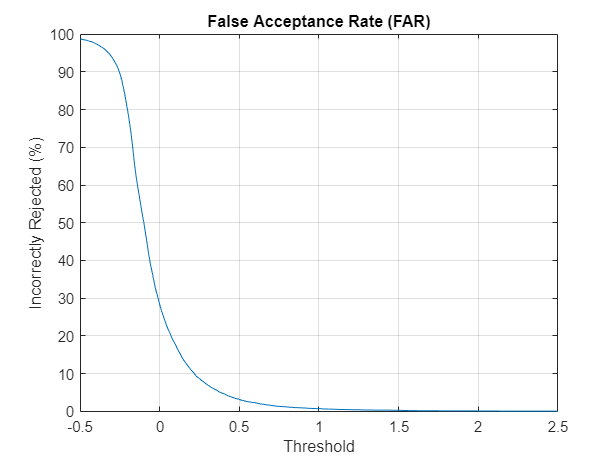As you move the threshold in a speaker verification system, you trade off between FAR and FRR. This is referred to as the detection error tradeoff (DET) and is commonly reported for binary classification problems.

```x1 = FAR*100; y1 = FRR*100; plot(x1,y1) grid on xlabel('False Acceptance Rate (%)') ylabel('False Rejection Rate (%)') title('Detection Error Tradeoff (DET) Curve')```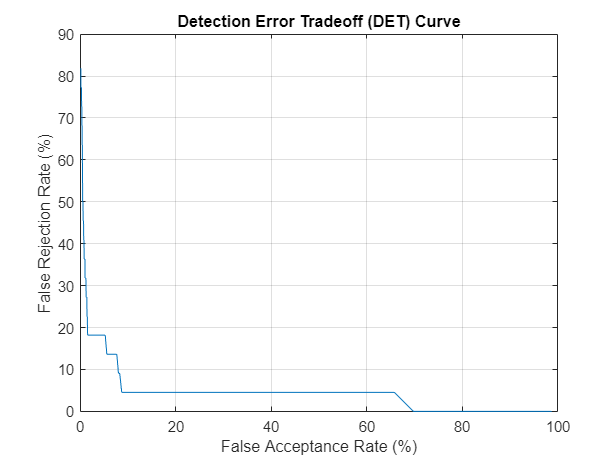#### Equal Error Rate (EER)

To compare multiple systems, you need a single metric that combines the FAR and FRR performances. For this, you determine the equal error rate (EER), which is the threshold where the FAR and FRR curves meet. In practice, the EER threshold may not be the best choice. For example, if speaker verification is used as part of a multi-authentication approach for wire transfers, FAR would most likely be weighed more heavily than FRR.

```[~,EERThresholdIdx] = min(abs(FAR - FRR)); EERThreshold = thresholds(EERThresholdIdx); EER = mean([FAR(EERThresholdIdx),FRR(EERThresholdIdx)]); plot(thresholds,FAR,'k', ... thresholds,FRR,'b', ... EERThreshold,EER,'ro','MarkerFaceColor','r') title(sprintf('Equal Error Rate = %0.2f, Threshold = %0.2f',EER,EERThreshold)) xlabel('Threshold') ylabel('Error Rate') legend('False Acceptance Rate (FAR)','False Rejection Rate (FRR)','Equal Error Rate (EER)') grid on```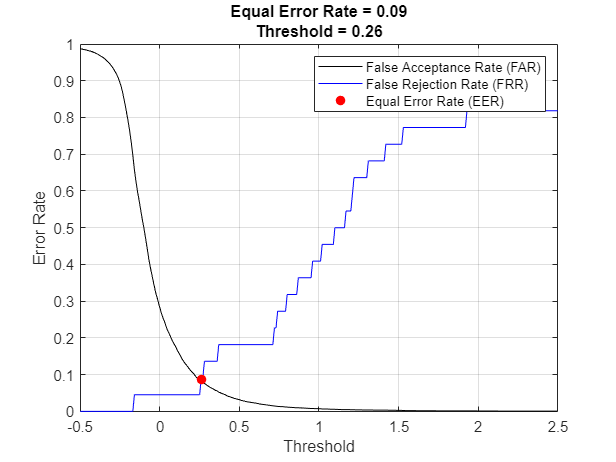If you changed parameters of the UBM training, consider resaving the MAT file with the new universal background model, `audioFeatureExtractor`, and norm factors.

```resave =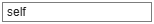false; if resave save('speakerVerificationExampleData.mat','ubm','afe','normFactors') end```

### Supporting Functions

#### Add User to Data Set

```function helperAddUser(fs,numToRecord,ID) % Create an audio device reader to read from your audio device deviceReader = audioDeviceReader('SampleRate',fs); % Initialize variables numRecordings = 1; audioIn = []; % Record the requested number while numRecordings <= numToRecord fprintf('Say "stop" once (recording %i of %i) ...',numRecordings,numToRecord) tic while toc<2 audioIn = [audioIn;deviceReader()]; end fprintf('complete.\n') idx = detectSpeech(audioIn,fs); if isempty(idx) fprintf('Speech not detected. Try again.\n') else audiowrite(sprintf('%s_%i.flac',ID,numRecordings),audioIn,fs) numRecordings = numRecordings+1; end pause(0.2) audioIn = []; end % Release the device release(deviceReader) end```

#### Enroll

```function speakerGMM = helperEnroll(ubm,afe,normFactors,adsEnroll) % Initialization numComponents = numel(ubm.ComponentProportion); numFeatures = size(ubm.mu,1); N = zeros(1,numComponents); F = zeros(numFeatures,numComponents); S = zeros(numFeatures,numComponents); NumFrames = 0; while hasdata(adsEnroll) % Read from the enrollment datastore audioData = read(adsEnroll); % 1. Extract the features and apply feature normalization [features,numFrames] = helperFeatureExtraction(audioData,afe,normFactors); % 2. Calculate the a posteriori probability. Use it to determine the % sufficient statistics (the count, and the first and second moments) [n,f,s] = helperExpectation(features,ubm); % 3. Update the sufficient statistics N = N + n; F = F + f; S = S + s; NumFrames = NumFrames + numFrames; end % Create the Gaussian mixture model that maximizes the expectation speakerGMM = helperMaximization(N,F,S); % Adapt the UBM to create the speaker model. Use a relevance factor of 16, % as proposed in  relevanceFactor = 16; % Determine adaption coefficient alpha = N ./ (N + relevanceFactor); % Adapt the means speakerGMM.mu = alpha.*speakerGMM.mu + (1-alpha).*ubm.mu; % Adapt the variances speakerGMM.sigma = alpha.*(S./N) + (1-alpha).*(ubm.sigma + ubm.mu.^2) - speakerGMM.mu.^2; speakerGMM.sigma = max(speakerGMM.sigma,eps); % Adapt the weights speakerGMM.ComponentProportion = alpha.*(N/sum(N)) + (1-alpha).*ubm.ComponentProportion; speakerGMM.ComponentProportion = speakerGMM.ComponentProportion./sum(speakerGMM.ComponentProportion); end```

#### Verify

```function verificationStatus = helperVerify(audioData,afe,normFactors,speakerGMM,ubm,threshold) % Extract features x = helperFeatureExtraction(audioData,afe,normFactors); % Determine the log-likelihood the audio came from the GMM adapted to % the speaker post = helperGMMLogLikelihood(x,speakerGMM); Lspeaker = helperLogSumExp(post); % Determine the log-likelihood the audio came form the GMM fit to all % speakers post = helperGMMLogLikelihood(x,ubm); Lubm = helperLogSumExp(post); % Calculate the ratio for all frames. Apply a moving median filter % to remove outliers, and then take the mean across the frames llr = mean(movmedian(Lspeaker - Lubm,3)); if llr > threshold verificationStatus = true; else verificationStatus = false; end end```

#### Feature Extraction

```function [features,numFrames] = helperFeatureExtraction(audioData,afe,normFactors) % Normalize audioData = audioData/max(abs(audioData(:))); % Protect against NaNs audioData(isnan(audioData)) = 0; % Isolate speech segment % The dataset used in this example has one word per audioData, if more % than one is speech section is detected, just use the longest % detected. idx = detectSpeech(audioData,afe.SampleRate); if size(idx,1)>1 [~,seg] = max(idx(:,2) - idx(:,1)); else seg = 1; end audioData = audioData(idx(seg,1):idx(seg,2)); % Feature extraction features = extract(afe,audioData); % Feature normalization if ~isempty(normFactors) features = (features-normFactors.Mean')./normFactors.STD'; end features = features'; % Cepstral mean subtraction (for channel noise) if ~isempty(normFactors) features = features - mean(features,'all'); end numFrames = size(features,2); end```

#### Log-sum-exponent

```function y = helperLogSumExp(x) % Calculate the log-sum-exponent while avoiding overflow a = max(x,[],1); y = a + sum(exp(bsxfun(@minus,x,a)),1); end```

#### Expectation

```function [N,F,S,L] = helperExpectation(features,gmm) post = helperGMMLogLikelihood(features,gmm); % Sum the likelihood over the frames L = helperLogSumExp(post); % Compute the sufficient statistics gamma = exp(post-L)'; N = sum(gamma,1); F = features * gamma; S = (features.*features) * gamma; L = sum(L); end```

#### Maximization

```function gmm = helperMaximization(N,F,S) N = max(N,eps); gmm.ComponentProportion = max(N/sum(N),eps); gmm.mu = bsxfun(@rdivide,F,N); gmm.sigma = max(bsxfun(@rdivide,S,N) - gmm.mu.^2,eps); end```

#### Gaussian Multi-Component Mixture Log-Likelihood

```function L = helperGMMLogLikelihood(x,gmm) xMinusMu = repmat(x,1,1,numel(gmm.ComponentProportion)) - permute(gmm.mu,[1,3,2]); permuteSigma = permute(gmm.sigma,[1,3,2]); Lunweighted = -0.5*(sum(log(permuteSigma),1) + sum(bsxfun(@times,xMinusMu,(bsxfun(@rdivide,xMinusMu,permuteSigma))),1) + size(gmm.mu,1)*log(2*pi)); temp = squeeze(permute(Lunweighted,[1,3,2])); if size(temp,1)==1 % If there is only one frame, the trailing singleton dimension was % removed in the permute. This accounts for that edge case temp = temp'; end L = bsxfun(@plus,temp,log(gmm.ComponentProportion)'); end```

### References